Check Full Chapter Explained - Continuity and Differentiability - Continuity and Differentiability Class 12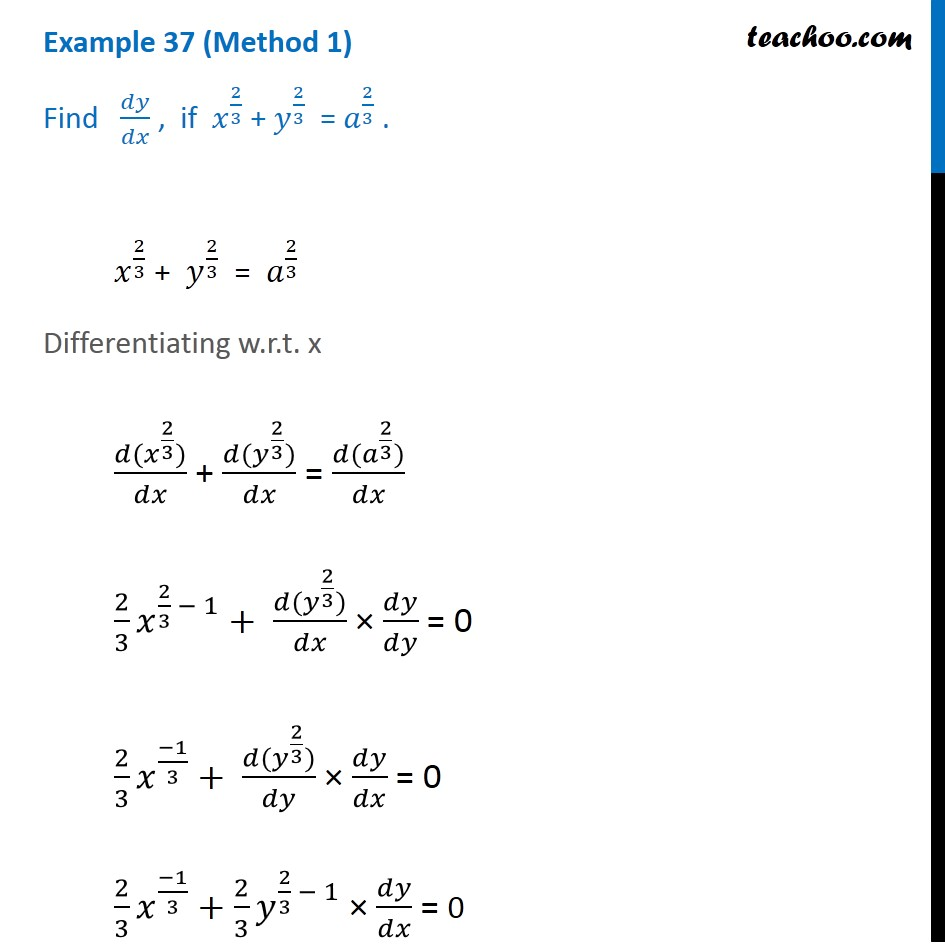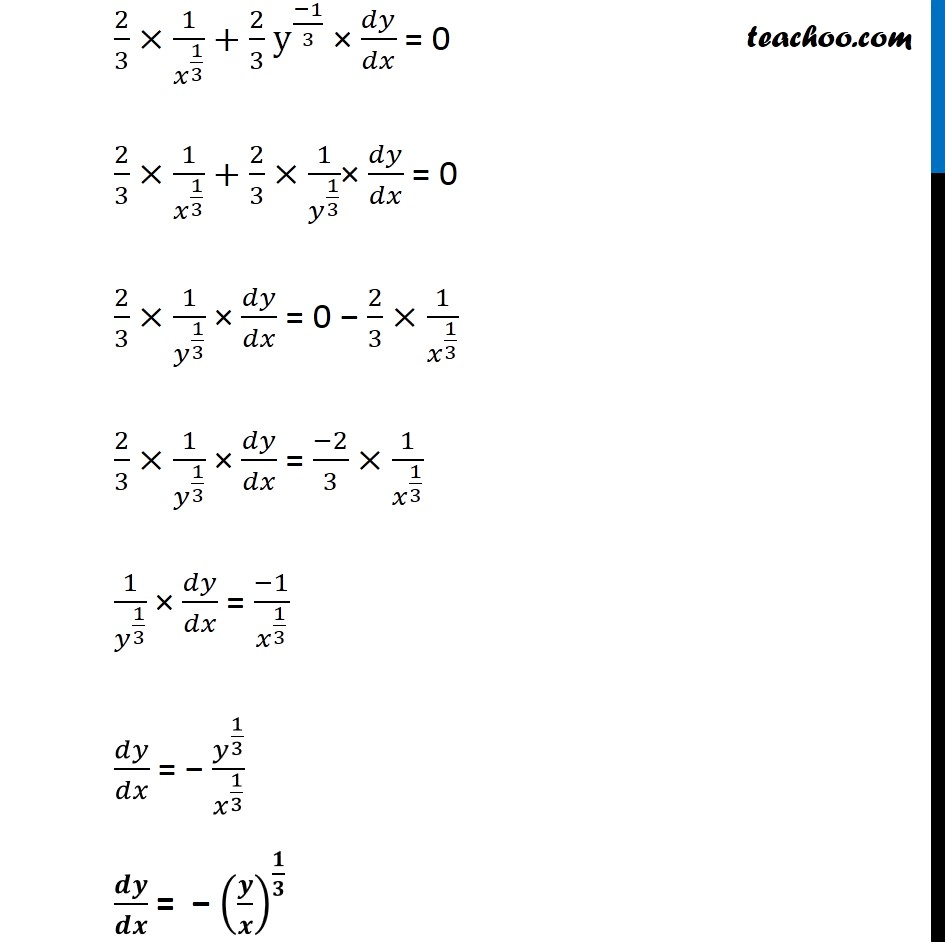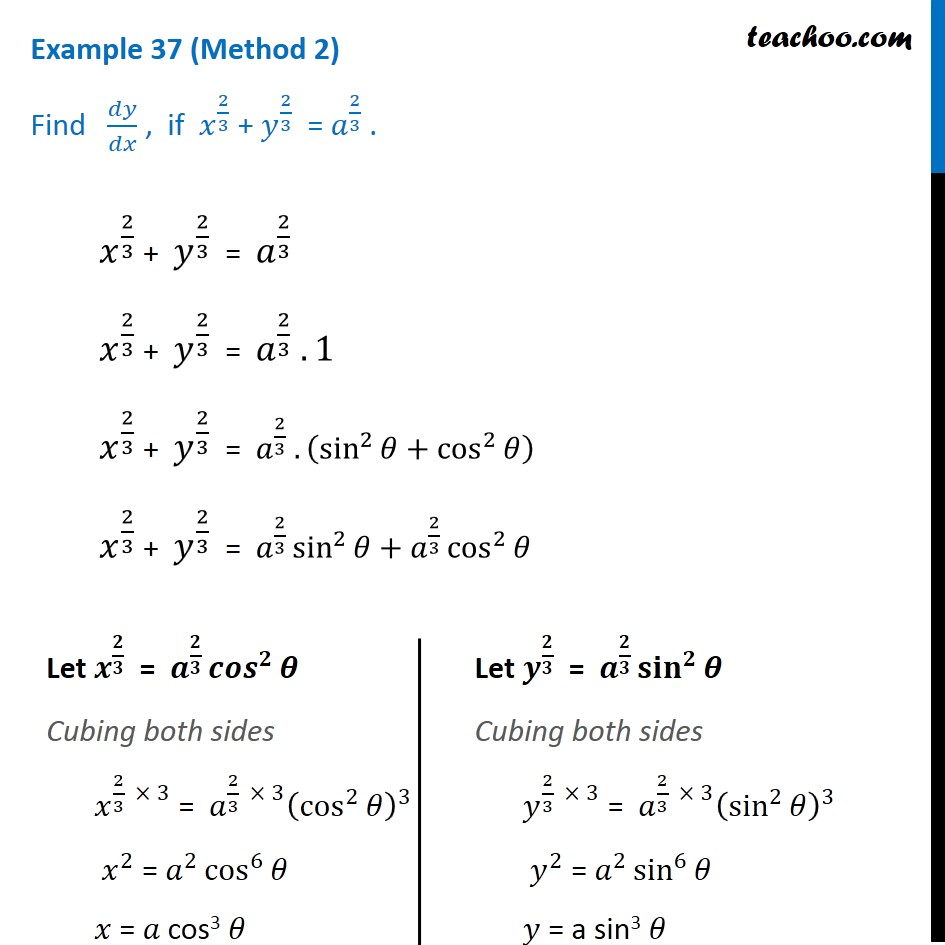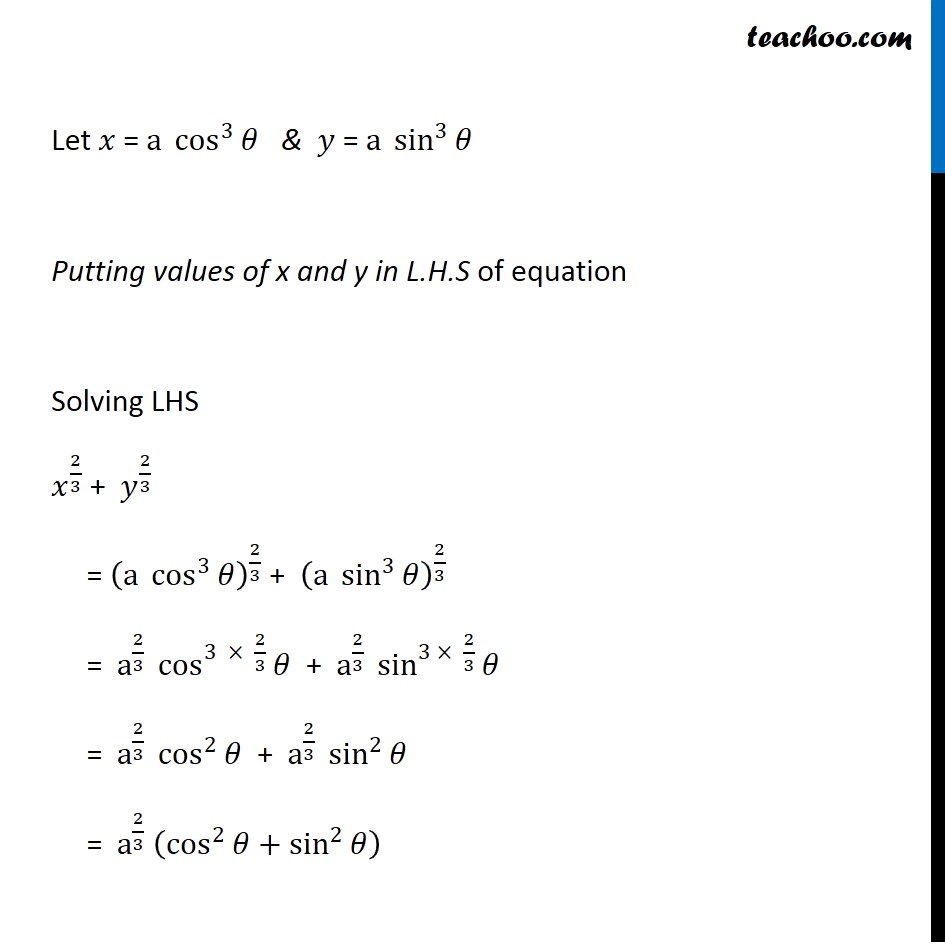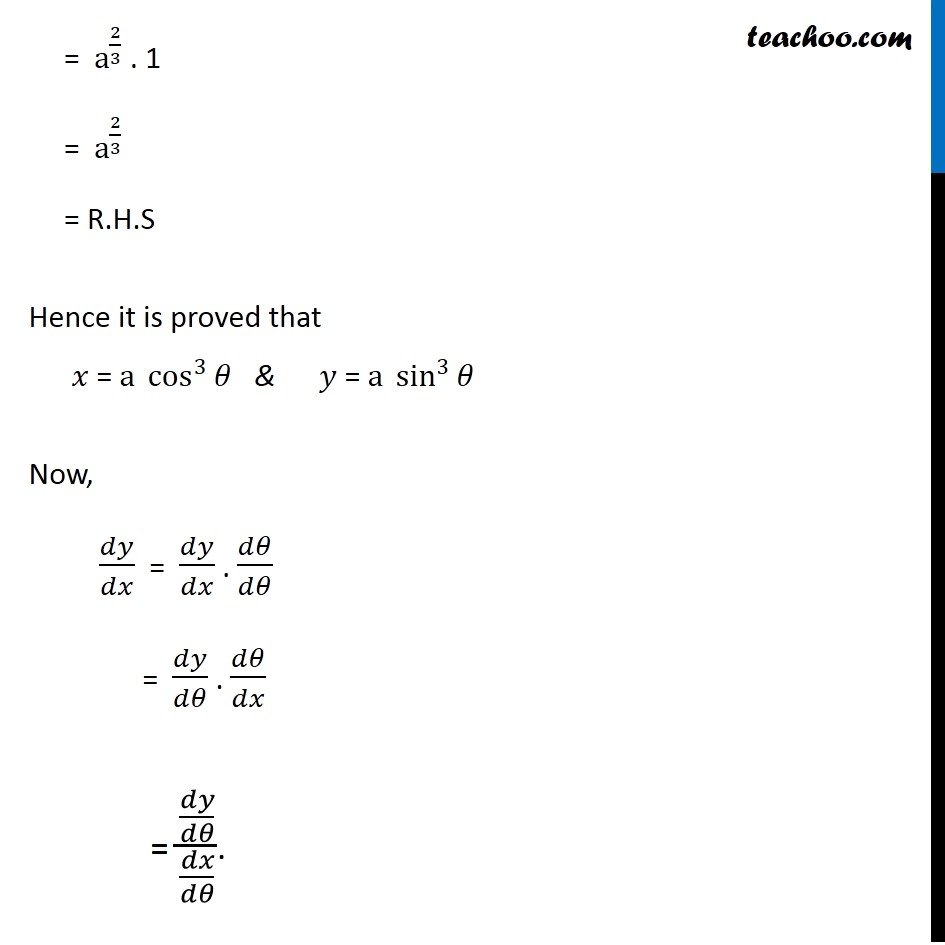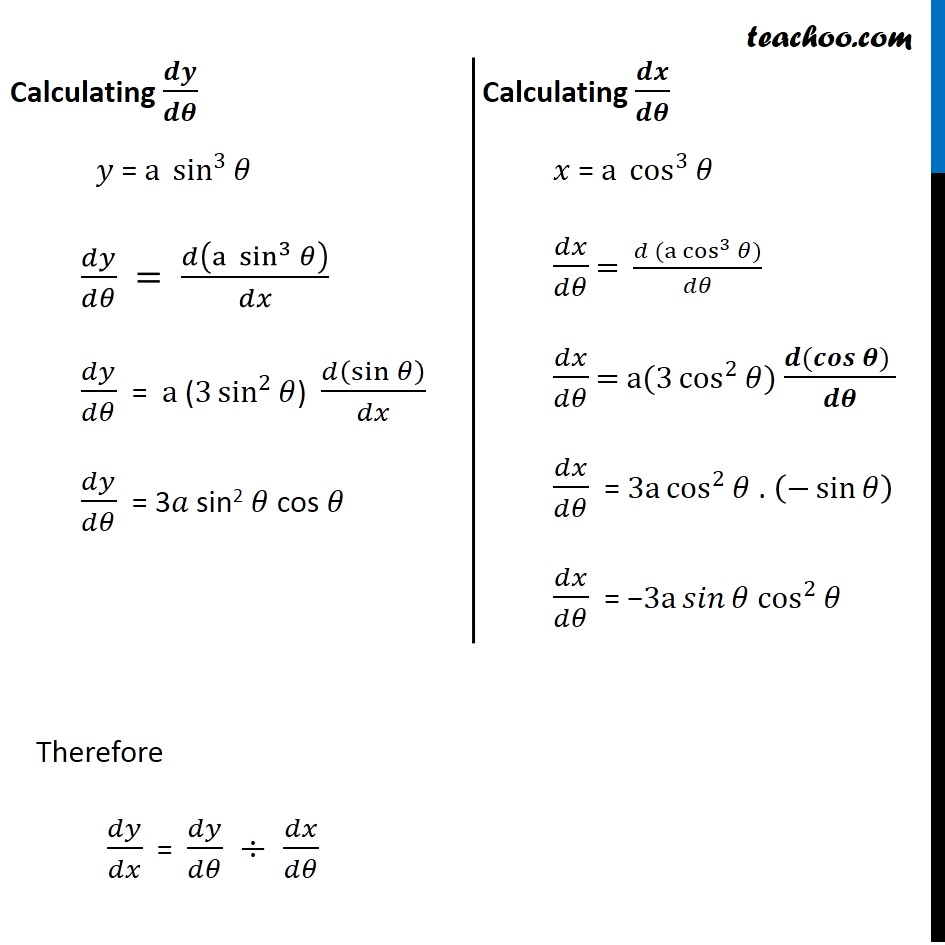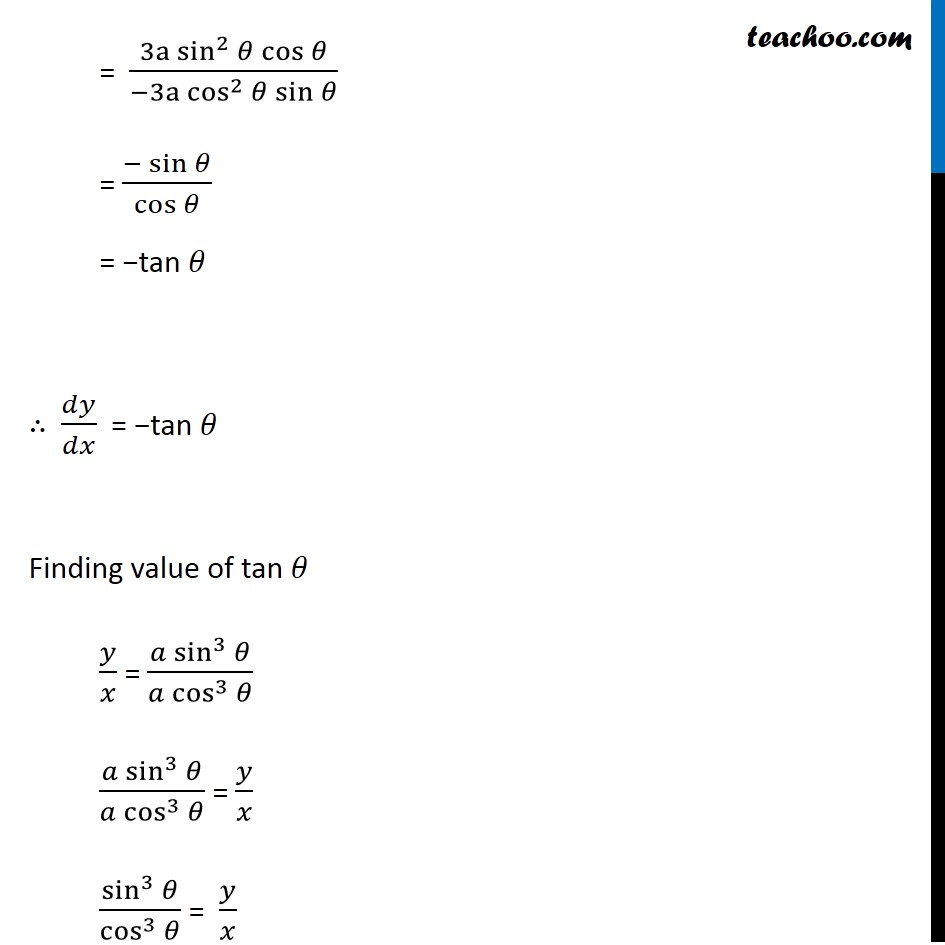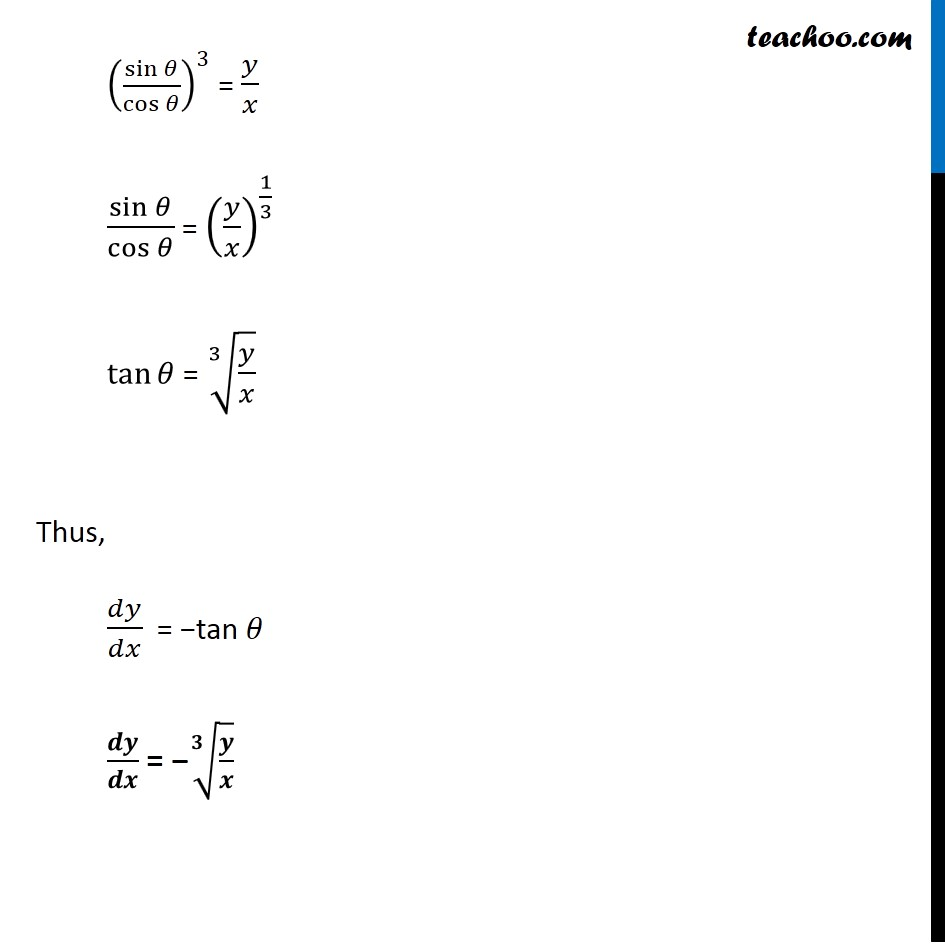1. Chapter 5 Class 12 Continuity and Differentiability
2. Serial order wise
3. Examples

Transcript

Example 37 (Method 1) Find 𝑑𝑦/𝑑𝑥 , if 𝑥^(2/3) + 𝑦^(2/3) = 𝑎^(2/3) . 𝑥^(2/3) + 𝑦^(2/3) = 𝑎^(2/3) Differentiating w.r.t. x (𝑑(𝑥^(2/3)))/𝑑𝑥 + (𝑑(𝑦^(2/3)))/𝑑𝑥 = (𝑑(𝑎^(2/3)))/𝑑𝑥 2/3 𝑥^(2/3 − 1) + (𝑑(𝑦^(2/3)))/𝑑𝑥 × 𝑑𝑦/𝑑𝑦 = 0 2/3 𝑥^((−1)/3) + (𝑑(𝑦^(2/3)))/𝑑𝑦 × 𝑑𝑦/𝑑𝑥 = 0 2/3 𝑥^((−1)/3) + 2/3 𝑦^(2/3 − 1) × 𝑑𝑦/𝑑𝑥 = 0 2/3×1/𝑥^(1/3) + 2/3 y^((−1)/3) × 𝑑𝑦/𝑑𝑥 = 0 2/3×1/𝑥^(1/3) + 2/3 × 1/𝑦^(1/3) × 𝑑𝑦/𝑑𝑥 = 0 2/3×1/𝑦^(1/3) × 𝑑𝑦/𝑑𝑥 = 0 − 2/3× 1/𝑥^(1/3) 2/3×1/𝑦^(1/3) × 𝑑𝑦/𝑑𝑥 = (−2)/3×1/𝑥^(1/3) 1/𝑦^(1/3) × 𝑑𝑦/𝑑𝑥 = (−1)/𝑥^(1/3) 𝑑𝑦/𝑑𝑥 = − 𝑦^(1/3)/𝑥^(1/3) 𝒅𝒚/𝒅𝒙 = − (𝒚/𝒙)^(𝟏/𝟑) Example 37 (Method 2) Find 𝑑𝑦/𝑑𝑥 , if 𝑥^(2/3) + 𝑦^(2/3) = 𝑎^(2/3) . 𝑥^(2/3) + 𝑦^(2/3) = 𝑎^(2/3) 𝑥^(2/3) + 𝑦^(2/3) = 𝑎^(2/3) . 1 𝑥^(2/3) + 𝑦^(2/3) = 𝑎^(2/3) . (sin^2⁡𝜃+cos^2⁡𝜃 ) 𝑥^(2/3) + 𝑦^(2/3) = 𝑎^(2/3) sin^2⁡𝜃+𝑎^(2/3) cos^2⁡𝜃 Let 𝒙^(𝟐/𝟑) = 𝒂^(𝟐/𝟑) 〖𝒄𝒐𝒔〗^𝟐⁡𝜽 Cubing both sides 𝑥^(2/3 \ × 3) = 𝑎^(2/3 × 3) (cos^2⁡𝜃 )^3 𝑥^2 = 𝑎^2 cos^6⁡𝜃 𝑥 = 𝑎 cos3 𝜃 Let 𝒚^(𝟐/𝟑) = 𝒂^(𝟐/𝟑) 〖𝐬𝐢𝐧〗^𝟐⁡𝜽 Cubing both sides 𝑦^(2/3 \ × 3) = 𝑎^(2/3 × 3) (sin^2⁡𝜃 )^3 𝑦^2 = 𝑎^2 sin^6⁡𝜃 𝑦 = a sin3 𝜃 Let 𝑥 = a cos^3⁡𝜃 & 𝑦 = a sin^3⁡𝜃 Putting values of x and y in L.H.S of equation Solving LHS 𝑥^(2/3) + 𝑦^(2/3) = (a cos^3⁡𝜃 )^(2/3) + (a sin^3⁡𝜃 )^(2/3) = a^(2/3) cos^(3 × 2/3)⁡𝜃 + a^(2/3) sin^(3 × 2/3)⁡𝜃 = a^(2/3) cos^2⁡𝜃 + a^(2/3) sin^2⁡𝜃 = a^(2/3) (cos^2⁡𝜃+sin^2⁡𝜃 ) = a^(2/3) . 1 = a^(2/3) = R.H.S Hence it is proved that 𝑥 = a cos^3⁡𝜃 & 𝑦 = a sin^3⁡𝜃 Now, 𝑑𝑦/𝑑𝑥 = 𝑑𝑦/𝑑𝑥 . 𝑑𝜃/𝑑𝜃 = 𝑑𝑦/𝑑𝜃 . 𝑑𝜃/𝑑𝑥 = (𝑑𝑦/𝑑𝜃)/(𝑑𝑥/𝑑𝜃). Calculating 𝒅𝒚/𝒅𝜽 𝑦 = a sin^3⁡𝜃 𝑑𝑦/𝑑𝜃 = 𝑑(a sin^3⁡𝜃 )/𝑑𝑥 𝑑𝑦/𝑑𝜃 = a (3 sin^2⁡𝜃) 𝑑(sin⁡𝜃 )/𝑑𝑥 𝑑𝑦/𝑑𝜃 = 3𝑎 sin2 𝜃 cos 𝜃 Calculating 𝒅𝒙/𝒅𝜽 𝑥 = a cos^3⁡𝜃 𝑑𝑥/𝑑𝜃 = (𝑑 (〖a cos〗^3⁡〖𝜃)〗)/𝑑𝜃 𝑑𝑥/𝑑𝜃 = a(3 cos^2⁡𝜃) (𝒅(𝒄𝒐𝒔 𝜽) )/𝒅𝜽 𝑑𝑥/𝑑𝜃 = 3a cos^2⁡𝜃 . (−sin⁡𝜃 ) 𝑑𝑥/𝑑𝜃 = −3a 𝑠𝑖𝑛⁡𝜃 cos^2⁡𝜃 Therefore 𝑑𝑦/𝑑𝑥 = 𝑑𝑦/𝑑𝜃 ÷ 𝑑𝑥/𝑑𝜃 = (3a sin^2⁡𝜃 cos⁡𝜃)/(−3a cos^2⁡𝜃 sin⁡𝜃 ) = (−sin⁡𝜃)/cos⁡𝜃 = −tan 𝜃 ∴ 𝑑𝑦/𝑑𝑥 = −tan 𝜃 Finding value of tan 𝜃 𝑦/𝑥 = (𝑎 sin^3⁡𝜃)/(𝑎 cos^3⁡𝜃 ) (𝑎 sin^3⁡𝜃)/(𝑎 cos^3⁡𝜃 ) = 𝑦/𝑥 sin^3⁡𝜃/cos^3⁡𝜃 = 𝑦/𝑥 (sin⁡𝜃/cos⁡𝜃 )^3 = 𝑦/𝑥 sin⁡𝜃/cos⁡𝜃 = (𝑦/𝑥)^(1/3) tan⁡𝜃 = ∛(𝑦/𝑥) Thus, 𝑑𝑦/𝑑𝑥 = −tan 𝜃 𝒅𝒚/𝒅𝒙 = −√(𝟑&𝒚/𝒙)

Examples